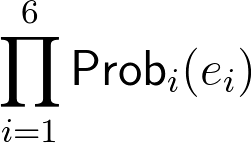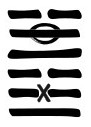# Events and ProbabilitesThe relevance of randomness in divination as been debated at length. One side of the camp claims that the divination process, for its very nature, reveals a deeper connection with the structure of reality and, for this very reason, the response is not random but determined by the moment in which the divination process occurs.
The other side of the camp responds that nothing can escape the law of physics and that reality itself seems to be governed, at quantum level, by randomness. Hence the statistics related to the divination process play a huge role.

I tend to agree with the latter view: the divination process, to me, is fully governed by the law of physics; the randomness of each method is key in interpreting the results. The probability to get a certain moving line, especially when multiple changing lines are present, is a factor to be taken into account. Getting six moving yin line is near to impossible with the the yarrow stalk method (i.e. possible but highly unlikely to happen), while using the dutch sticks method is as probable as any other outcome. You need to be aware of these differences to correctly interpret the response.

Any method for casting I Ching hexagrams can be characterized, at the most abstract level, by the  probability it assigns to the each possible response.

#### Line probabilities

The most used methods build the hexagram one line at the time (usually from bottom to top); it is, hence, important to consider the probability assigned to each line.
We will use Probi (e) to signify the "probability that the event e will happen at line i". In the majority of cases the line i is not significant and will be omitted.
For example for the three coins method, for which the probabilities do not depend on the line, the indication Prob(7) = 3/8 means that a non movin yang line () has 3 out of 8 chances to occur in any position.

#### Response probabilitesGiven the line probabilities, the probability to get a specific response (out of the 4096 which are possible) is given by the formula on the left (i.e. by the multiplication of the probabilities of each line) where ei is the type of line i  (6,7,8,9).For eaxample, the probability to get the hexagram on the right as response is (counting from bottom to top): Prob1(7) * Prob2(6) * Prob3(8) * Prob4(7) * Prob5(9) * Prob6(8)

For the three coins method it would be:
3/8 * 1/8 * 3/8 * 3/8 * 1/8 * 3/881/262144 = 0.031%

For the yarrow stalks method it would be:
5/16 * 1/16 * 7/16 * 5/16 * 3/16 * 7/163675/16777216 = 0.022%

If we want to calculate the probability of getting one of the 64 hexagrams (regardless of moving lines) as response, the formula in the previous paragraph is still applicable. The difference is that ei represent the fact that the line is either yin or a yang line:
Probi(yin) = Probi(6) + Probi(8)
Probi(yang) = Probi(9) + Probi(7)

Note that if  the probability is independent of the line and Prob(yin) = Prob(yang) = 1/2  (as it is often the case) the formula above returns 1/26 = 1/64 which means that each hexagram is equiprobable.

Some simple formula for the most common cases (and with the most common assumptions):

Probability of getting a response with n yin lines:         [Prob(6) + Prob(8)]n
Probability of getting a response with n yang lines:      [Prob(9) + Prob(7)]n
Probability of getting a response with n moving lines:  [Prob(6) + Prob(9)]n

#### Secondary Hexagram

To determine the probability to get a given hexagram as the secondary one we can consider that a secondary hexagram contains a yang line in position i in two cases: if the primary hexagram contains a yang line in that position or if the primary hexgram has a moving yin line in that position; and similarly for a yin line.
Probi(secondary_yin) = Probi(9) + Probi(8)
Probi(secondary_yang) = Probi(6) + Probi(7)

Only if the method is symmetric (i.e. [Prob(6) = Prob(9)] and [Prob(7) = Prob(8)]) each hexagram will have the same probability of being the secondary hexagram.